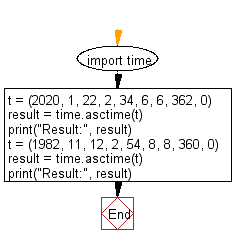﻿ Python: A tuple containing 9 elements corresponding to structure time as an argument and returns a string representing it - w3resource# Python: A tuple containing 9 elements corresponding to structure time as an argument and returns a string representing it

## Python Datetime: Exercise-62 with Solution

Write a Python program that takes a tuple containing 9 elements corresponding to structure time as an argument and returns a string representing it.

Sample Solution:

Python Code:

``````import time
t = (2020, 1, 22, 2, 34, 6, 6, 362, 0)
result = time.asctime(t)
print("Result:", result)
t = (1982, 11, 12, 2, 54, 8, 8, 360, 0)
result = time.asctime(t)
print("Result:", result)
```
```

Sample Output:

```Result: Sun Jan 22 02:34:06 2020
Result: Tue Nov 12 02:54:08 1982
```

Flowchart:## Visualize Python code execution:

The following tool visualize what the computer is doing step-by-step as it executes the said program:

Python Code Editor:

What is the difficulty level of this exercise?

Test your Programming skills with w3resource's quiz.

﻿

```>>> a = ['j', 'a', 'k', 'd', 'c']